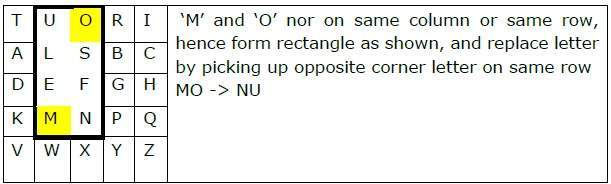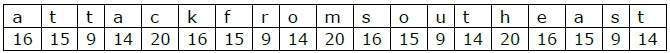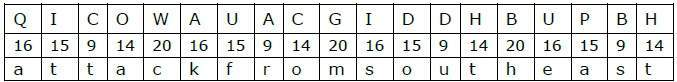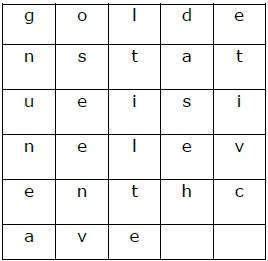In the second chapter, we discussed the fundamentals of modern cryptography. We equated cryptography with a toolkit where various cryptographic techniques are considered as the basic tools. One of these tools is the Symmetric Key Encryption where the key used for encryption and decryption is the same.

In this chapter, we discuss this technique further and its applications to develop various cryptosystems.

## Earlier Cryptographic Systems

Before proceeding further, you need to know some facts about historical cryptosystems −

• All of these systems are based on symmetric key encryption scheme.

• The only security service these systems provide is confidentiality of information.

• Unlike modern systems which are digital and treat data as binary numbers, the earlier systems worked on alphabets as basic element.

These earlier cryptographic systems are also referred to as Ciphers. In general, a cipher is simply just a set of steps (an algorithm) for performing both an encryption, and the corresponding decryption.

## Caesar Cipher

It is a mono-alphabetic cipher wherein each letter of the plaintext is substituted by another letter to form the ciphertext. It is a simplest form of substitution cipher scheme.

This cryptosystem is generally referred to as the Shift Cipher. The concept is to replace each alphabet by another alphabet which is ‘shifted’ by some fixed number between 0 and 25.

For this type of scheme, both sender and receiver agree on a ‘secret shift number’ for shifting the alphabet. This number which is between 0 and 25 becomes the key of encryption.

The name ‘Caesar Cipher’ is occasionally used to describe the Shift Cipher when the ‘shift of three’ is used.

### Process of Shift Cipher

• In order to encrypt a plaintext letter, the sender positions the sliding ruler underneath the first set of plaintext letters and slides it to LEFT by the number of positions of the secret shift.

• The plaintext letter is then encrypted to the ciphertext letter on the sliding ruler underneath. The result of this process is depicted in the following illustration for an agreed shift of three positions. In this case, the plaintext ‘tutorial’ is encrypted to the ciphertext ‘WXWRULDO’. Here is the ciphertext alphabet for a Shift of 3 −• On receiving the ciphertext, the receiver who also knows the secret shift, positions his sliding ruler underneath the ciphertext alphabet and slides it to RIGHT by the agreed shift number, 3 in this case.

• He then replaces the ciphertext letter by the plaintext letter on the sliding ruler underneath. Hence the ciphertext ‘WXWRULDO’ is decrypted to ‘tutorial’. To decrypt a message encoded with a Shift of 3, generate the plaintext alphabet using a shift of ‘-3’ as shown below −### Security Value

Caesar Cipher is not a secure cryptosystem because there are only 26 possible keys to try out. An attacker can carry out an exhaustive key search with available limited computing resources.

## Simple Substitution Cipher

It is an improvement to the Caesar Cipher. Instead of shifting the alphabets by some number, this scheme uses some permutation of the letters in alphabet.

For example, A.B…..Y.Z and Z.Y……B.A are two obvious permutation of all the letters in alphabet. Permutation is nothing but a jumbled up set of alphabets.

With 26 letters in alphabet, the possible permutations are 26! (Factorial of 26) which is equal to 4x1026. The sender and the receiver may choose any one of these possible permutation as a ciphertext alphabet. This permutation is the secret key of the scheme.

### Process of Simple Substitution Cipher

• Write the alphabets A, B, C,...,Z in the natural order.

• The sender and the receiver decide on a randomly selected permutation of the letters of the alphabet.

• Underneath the natural order alphabets, write out the chosen permutation of the letters of the alphabet. For encryption, sender replaces each plaintext letters by substituting the permutation letter that is directly beneath it in the table. This process is shown in the following illustration. In this example, the chosen permutation is K,D, G, ..., O. The plaintext ‘point’ is encrypted to ‘MJBXZ’.

Here is a jumbled Ciphertext alphabet, where the order of the ciphertext letters is a key.• On receiving the ciphertext, the receiver, who also knows the randomly chosen permutation, replaces each ciphertext letter on the bottom row with the corresponding plaintext letter in the top row. The ciphertext ‘MJBXZ’ is decrypted to ‘point’.

### Security Value

Simple Substitution Cipher is a considerable improvement over the Caesar Cipher. The possible number of keys is large (26!) and even the modern computing systems are not yet powerful enough to comfortably launch a brute force attack to break the system. However, the Simple Substitution Cipher has a simple design and it is prone to design flaws, say choosing obvious permutation, this cryptosystem can be easily broken.

## Monoalphabetic and Polyalphabetic Cipher

Monoalphabetic cipher is a substitution cipher in which for a given key, the cipher alphabet for each plain alphabet is fixed throughout the encryption process. For example, if ‘A’ is encrypted as ‘D’, for any number of occurrence in that plaintext, ‘A’ will always get encrypted to ‘D’.

All of the substitution ciphers we have discussed earlier in this chapter are monoalphabetic; these ciphers are highly susceptible to cryptanalysis.

Polyalphabetic Cipher is a substitution cipher in which the cipher alphabet for the plain alphabet may be different at different places during the encryption process. The next two examples, playfair and Vigenere Cipher are polyalphabetic ciphers.

## Playfair Cipher

In this scheme, pairs of letters are encrypted, instead of single letters as in the case of simple substitution cipher.

In playfair cipher, initially a key table is created. The key table is a 5×5 grid of alphabets that acts as the key for encrypting the plaintext. Each of the 25 alphabets must be unique and one letter of the alphabet (usually J) is omitted from the table as we need only 25 alphabets instead of 26. If the plaintext contains J, then it is replaced by I.

The sender and the receiver deicide on a particular key, say ‘tutorials’. In a key table, the first characters (going left to right) in the table is the phrase, excluding the duplicate letters. The rest of the table will be filled with the remaining letters of the alphabet, in natural order. The key table works out to be −### Process of Playfair Cipher

• First, a plaintext message is split into pairs of two letters (digraphs). If there is an odd number of letters, a Z is added to the last letter. Let us say we want to encrypt the message “hide money”. It will be written as −

HI DE MO NE YZ

• The rules of encryption are −

• If both the letters are in the same column, take the letter below each one (going back to the top if at the bottom)

•  T U O R I ‘H’ and ‘I’ are in same column, hence take letter below them to replace. HI → QC A L S B C D E F G H K M N P Q V W X Y Z
• If both letters are in the same row, take the letter to the right of each one (going back to the left if at the farthest right)

•  T U O R I ‘D’ and ‘E’ are in same row, hence take letter to the right of them to replace. DE → EF A L S B C D E F G H K M N P Q V W X Y Z
• If neither of the preceding two rules are true, form a rectangle with the two letters and take the letters on the horizontal opposite corner of the rectangle.Using these rules, the result of the encryption of ‘hide money’ with the key of ‘tutorials’ would be −

QC EF NU MF ZV

Decrypting the Playfair cipher is as simple as doing the same process in reverse. Receiver has the same key and can create the same key table, and then decrypt any messages made using that key.

### Security Value

It is also a substitution cipher and is difficult to break compared to the simple substitution cipher. As in case of substitution cipher, cryptanalysis is possible on the Playfair cipher as well, however it would be against 625 possible pairs of letters (25x25 alphabets) instead of 26 different possible alphabets.

The Playfair cipher was used mainly to protect important, yet non-critical secrets, as it is quick to use and requires no special equipment.

## Vigenere Cipher

This scheme of cipher uses a text string (say, a word) as a key, which is then used for doing a number of shifts on the plaintext.

For example, let’s assume the key is ‘point’. Each alphabet of the key is converted to its respective numeric value: In this case,

p → 16, o → 15, i → 9, n → 14, and t → 20.

Thus, the key is: 16 15 9 14 20.

### Process of Vigenere Cipher

• The sender and the receiver decide on a key. Say ‘point’ is the key. Numeric representation of this key is ‘16 15 9 14 20’.

• The sender wants to encrypt the message, say ‘attack from south east’. He will arrange plaintext and numeric key as follows −• He now shifts each plaintext alphabet by the number written below it to create ciphertext as shown below −• Here, each plaintext character has been shifted by a different amount – and that amount is determined by the key. The key must be less than or equal to the size of the message.

• For decryption, the receiver uses the same key and shifts received ciphertext in reverse order to obtain the plaintext.### Security Value

Vigenere Cipher was designed by tweaking the standard Caesar cipher to reduce the effectiveness of cryptanalysis on the ciphertext and make a cryptosystem more robust. It is significantly more secure than a regular Caesar Cipher.

In the history, it was regularly used for protecting sensitive political and military information. It was referred to as the unbreakable cipher due to the difficulty it posed to the cryptanalysis.

### Variants of Vigenere Cipher

There are two special cases of Vigenere cipher −

• The keyword length is same as plaintect message. This case is called Vernam Cipher. It is more secure than typical Vigenere cipher.

• Vigenere cipher becomes a cryptosystem with perfect secrecy, which is called One-time pad.

The circumstances are −

• The length of the keyword is same as the length of the plaintext.
• The keyword is a randomly generated string of alphabets.
• The keyword is used only once.

### Security Value

Let us compare Shift cipher with one-time pad.

### Shift Cipher − Easy to Break

In case of Shift cipher, the entire message could have had a shift between 1 and 25. This is a very small size, and very easy to brute force. However, with each character now having its own individual shift between 1 and 26, the possible keys grow exponentially for the message.

### One-time Pad − Impossible to Break

Let us say, we encrypt the name “point” with a one-time pad. It is a 5 letter text. To break the ciphertext by brute force, you need to try all possibilities of keys and conduct computation for (26 x 26 x 26 x 26 x 26) = 265 = 11881376 times. That’s for a message with 5 alphabets. Thus, for a longer message, the computation grows exponentially with every additional alphabet. This makes it computationally impossible to break the ciphertext by brute force.

## Transposition Cipher

It is another type of cipher where the order of the alphabets in the plaintext is rearranged to create the ciphertext. The actual plaintext alphabets are not replaced.

An example is a ‘simple columnar transposition’ cipher where the plaintext is written horizontally with a certain alphabet width. Then the ciphertext is read vertically as shown.

For example, the plaintext is “golden statue is in eleventh cave” and the secret random key chosen is “five”. We arrange this text horizontally in table with number of column equal to key value. The resulting text is shown below.The ciphertext is obtained by reading column vertically downward from first to last column. The ciphertext is ‘gnuneaoseenvltiltedasehetivc’.

To decrypt, the receiver prepares similar table. The number of columns is equal to key number. The number of rows is obtained by dividing number of total ciphertext alphabets by key value and rounding of the quotient to next integer value.

The receiver then writes the received ciphertext vertically down and from left to right column. To obtain the text, he reads horizontally left to right and from top to bottom row.